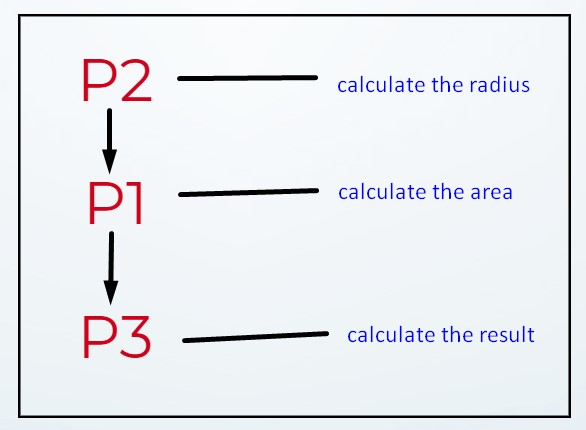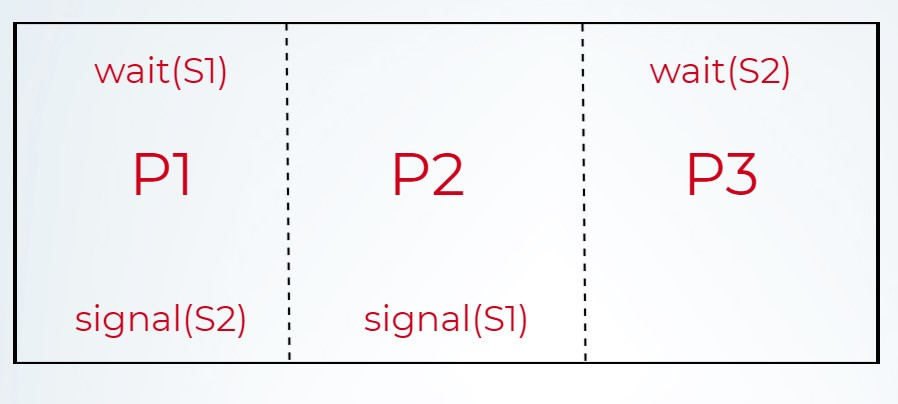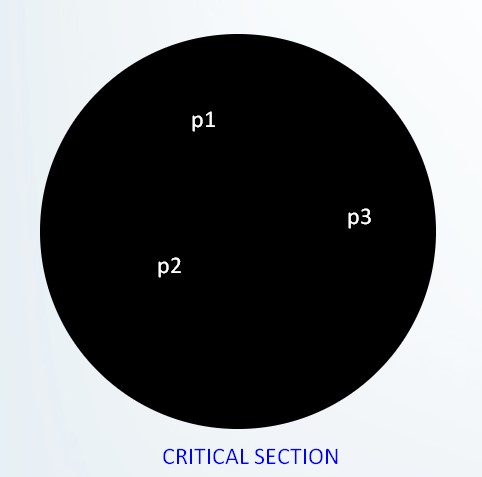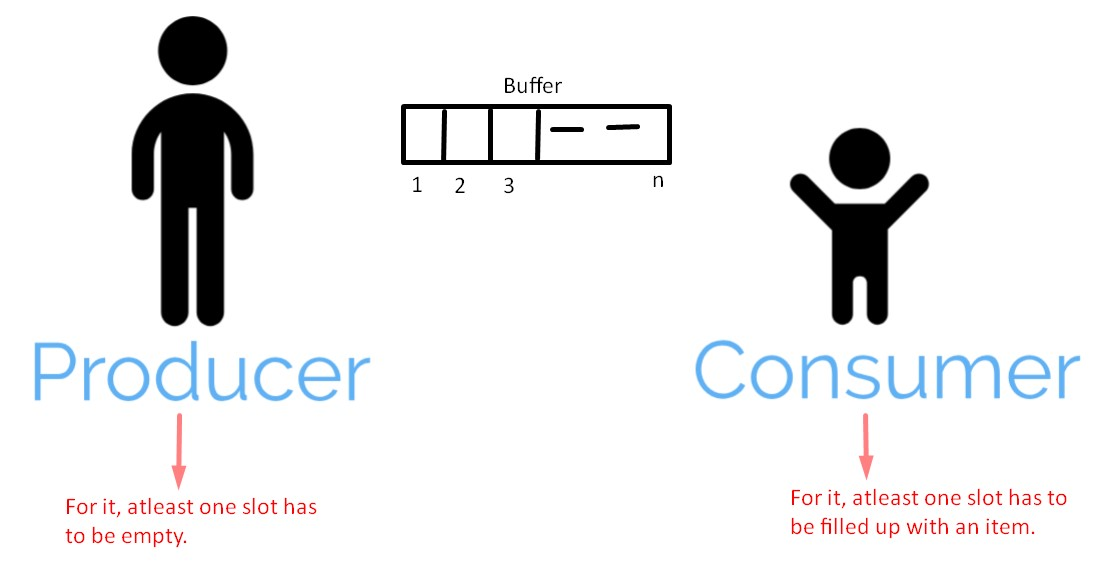How To

# What is a semaphore and what problems it helps to solve?## Semaphore

A Semaphore is nothing, but an integer variable (say “S”) that after initialization; it can only be accessed by two standard atomic operations:

wait(S): Its main task is to decrease the value of the semaphore variable by “1” and the code for the wait(S) is as follows:

``````wait(S)
{
while(S <= 0); // this while loop has no body

S = S - 1;
}

``````

There is a trick in the code for the wait(S) as for the value of S = 1, while is executed and the condition results as a false then after that S = S – 1 is executed and the value of S is decremented by 1.

But when the value of S = 0 then while’s condition is checked and results as a true, but the trick here is that even after the while condition resulted as a true result but because of the semi-colon (;) written after the while loop and there is no loop body for the while loop then, as a result, the control will never gets to go next and it will eventually get stuck in an infinite loop (Busy Waiting).

signal(S): Its main task is to increase the value of the semaphore variable by “1” and the code for the signal(S) is as follows:

``````signal(S)
{

S = S + 1;

}``````

The code for the signal(S) is simple to understand as its only work is to increment the value of the semaphore variable by 1 and nothing else. However, it can be understood that the code for the wait(S) is more complex than this.

Semaphore variable or variables (in a case when we are using more than one semaphore variable to solve a problem) helps us in achieving an N-process solution.

A Semaphore variable can be used to solve the following:

1. Critical Section problem
2. Deciding order of execution of processes
3. Managing Resources
4. Producer-Consumer problem

## Semaphore for the “critical section problem”

The code for the problem is as follows:

``````do {

wait(S);

// critical section

signal(S);

} while(condition)``````

Whenever a process wants to enter into the critical section, it has to first execute wait(S) and enters into the critical section. Now, because of wait(S) executed by a process, if another process tries to enter into the critical section with an attempt to execute wait(S) it will not be able to get out of while loop of wait(S) because of S‘s current value i.e. S = 0 (set by a previous process, which is now in the critical section).

And because of that, no other process can enter into the critical section until and unless the value of Semaphore S will become 1 again (S = 1) which is initially set to S = 0 by the previous process which is currently in the critical section as discussed above.

Note: All the switching of processes is known as context-switching.

## Semaphore for deciding the “order of execution of processes”

There can be N-processes in the real world and sometimes we want to perform tasks on order basis i.e. we want to calculate one thing first and another thing next and one after that and we want to make them execute them in a particular order.This can also be done by the Operating System with the help of scheduling algorithms, but sometimes we need a specific order of execution for some cases and for that semaphore is used.

Initial values for the semaphores are S1 = 0 and S2 = 0Now, our task is to run the P2 first in order to compute the radius before we go for P1 which is going to calculate the area and P3 for the result at the end. So, to achieve that, we have used two Semaphores S1 and S2, now the code for P1 will not be executed because of the wait(S1) before it and as the initial value for S1 = 0 then it will not be able to get out of while loop of wait(S) because of S1‘s current value i.e. S1 = 0 and same is the case with P3 for wait(S2) and after P1 and P3 only P2 is the one which does not have a wait(S) to execute and as a result of that it will enter the critical section without any problem. After that, as the signal(S1) code is executed by P2 after coming out of the critical section and signal() is only executed for S1 and only P1 has a wait(S1) code so next in the row is P1 after the completion of P1 it will set the signal for S2 by signal(S2) and from above we can see that only P3 has a wait(S2), so last to be executed is P3.

In this way, by using the semaphore like this we can decide the execution of the order of statements.

## Semaphore for “managing resources”

There are situations when we want to manage the resources but the right method is not known, this problem can be easily solved with the help of semaphores.

For example, we have 3 printers and 3 processes then we want 3 processes to be able to access those printers without any problem and whenever a 4th process wants to access the printer, it should not be allowed, as there are only 3 printers available at the moment and all of them are currently in use.Let us say, the initial value for a Semaphore S (say) is S = 3, now whenever a process enters to the critical section (which is a pool of printers here) then the value of S is decreased by 1 as wait(S) is written before the critical section and similarly after all the 3 processes executed the wait(S), now the value of S at the moment is S = 0, when all the 3 processes are in the critical section. However, if a 4th process tries to enter into the critical section, it will eventually fail to do so, because of the current value of S = 0 and it will end up stuck into an infinite loop.

And in this way, we have stated that for an X number of resources of a particular type, only X number of processes can access them.

## Semaphore for “producer-consumer problem”

Question: What is the issue?

Answer: There is a buffer with “N-slots” and at a point of time, both producer and consumer are performing an action on one of the slots at the same time and as a result, there will be a case when a crash occurs. So, the N-slots becomes a critical section because we want at one time, only one of (producer or consumer) can access a slot at a point of time. Therefore, we need the semaphore to solve this problem.Let us consider 3 semaphore variables:

1. S = 1 => this is going to control that at one time only one from (producer or consumer) is going to access a single slot.
2. E = n => this will keep a track of “emplty slots”.
3. F = 0 => this will keep a track of “how many slots are full”.

Following is the code for Producer():

``````void producer()
{
while(True)
{
produce()
wait(E)
wait(S)
append()
signal(S)
signal(F)
}
}
``````

Explanation: Let us assume the initial values are S = 1, E = 3, F = 0. Now, whenever the producer runs the produce() function and produces an item, then it decrements the value of E by 1 (as this is what the code for the wait() tends to do) and by this, it is being told that out of 3 slots available earlier, now after one item is produced (inserted with the help of append()) only 2 slots are left. After that, the value for S is also decremented by 1 (initial value is 1) and this is done to make sure that while producer process is in the critical section no other process can enter into it. Now, when the producer is coming out of the critical section it executes signal(S) and again increments the value of S by 1 (thus giving a permission to other processes to enter into the critical section) and also signal(F) is executed for increment in the value of F by 1 (thus saying that, one slot is filled up now).

Note: Above explanation is the single/one iteration of producer code.

Following is the code for Consumer():

``````void consumer()
{
while(True)
{
wait(F)
wait(S)
take()
signal(S)
signal(E)
use()
}
}``````

Explanation: We will continue from the explanation of producer process and now, when one slot is filled up with an item produced by producer process then consumer process wants to consume that item and we need to make sure that no other process will interfere during that. So, in order to achieve that we have written a code for consumer process with semaphores used in it. Initially, the values are S = 1, E = 2, F = 1. Now, when wait(F) is executed from consumer process then the value of F is decremented by 1 (stating that no slot is full, all are empty as the value of F becomes 0) after that, wait(S) is executed and the value of S is decremented by 1 (stating that no other process can enter into the critical section). After that take() function is executing (stating that, now the consumer can consume the item produced earlier by the producer process) and after coming out of the critical section, signal(S) is executed and increments the value of S by 1 (stating that other processes can now enter into the critical section) and lastly, signal(E) is executed and increments the value of E by 1 (stating that, all the slots are empty now, because the value of E becomes 3 again which was the initial value).

Note: Above explanation is the single/one iteration of consumer code.

Question: So, What the problem actually is?

Answer: Let us say there is a document in the computer system and at a point of time more than one readers and writers are trying to access that particular document and as a result of this concurrent access, there may be a case of race condition. So, in order to remove that we have to take the help of semaphores. Moreover, there can be two cases for this:

1. For Readers: Any number of readers can read at a point of time, but any write operation should be not be performed during that.
2. For Writers: Whenever a writer is trying to write or currently writing to a document then, in that case, the writer should make sure that during that, no other reader as well a writer should not perform a read or a write operation and if not avoided it may lead to data inconsistent problem.In order to solve the problem mentioned/described above we have to use 3 semaphores:

1. wrt = This is going to help in blocking the reader as well as the writer.
2. mutex = This will help in synchronizing between different readers and is particularly used to avoid the clash between the processes when more than one is performing an action on the shared semaphore variable readcount.
3. readcount = This will keep a track of, how many readers are currently in the critical section.

Initially, values for the same are:

• wrt = 1
• mutex = 1

Following is the code for Reader:

``````wait(mutex)
if (readcount == 1)  // only run by first reader to block any writer
wait(wrt);
signal(mutex)

wait(mutex)
if (readcount == 0)  // only run by last reader to allow any writer
signal(wrt);
signal(mutex)``````

``````wait(wrt)